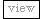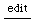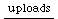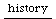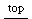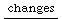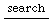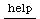## predicting series

### 1.

Given a growth rate and starting value, how many iters it takes to reach a certain value?
```problem:
start * pow(grow, iters) = end. find iters.

solution:
pow(grow, iters) = end / start. divide by start.
iters * log(grow) = log(end / start). take natural logs.
iters = log(end / start) / log(grow). divide by log(grow)
iters might not be an integer value!
```

### B.

Given a growth rate and starting value, how many iters it takes for the sum of the results to reach a certain value?
```..I just now figured this formula out experimentally.. bombs out if grow=1
sum(i=1,iters. of pow(grow,i)) = (pow(grow,iters) - 1) / (grow - 1)

problem:
start * ((pow(grow,iters) - 1) / (grow - 1)) = sum. find iters.

solution:
(pow(grow,iters) - 1) / (grow - 1) = sum / start. divide by start.
pow(grow,iters) - 1 = (grow - 1) * sum / start. mult by (grow - 1)
pow(grow,iters) = 1 + (grow - 1) * sum / start. add 1.
iters * log(grow) = log(1 + (grow - 1) * sum / start). take natural log.
iters = log(1 + (grow - 1) * sum / start) / log(grow). divide by log(grow).
iters might not be an integer value!
```

### III

Given a certain result value and a number of iterations, what should the growth rate be?
```problem:
start * pow(grow, iters) = end. find grow.

solution:
pow(grow, iters) = end / start. divide by start.
iters * log(grow) = log(end / start). take natural logs.
log(grow) = log(end / start) / iters. divide by iters.
grow = exp(log(end / start) / iters). exponentiate.
```

### Fourth:

Given a certain sum of the results and a number of iterations, what should the growth rate be?
```problem:
start * ((pow(grow,iters) - 1) / (grow - 1)) = sum. find grow.

solution:
(pow(grow,iters) - 1) / (grow - 1) = sum / start. divide by start.
```

hmm this one gets more involved.. I'll leave it for now..
• — james mccartney (quoted from a mail)